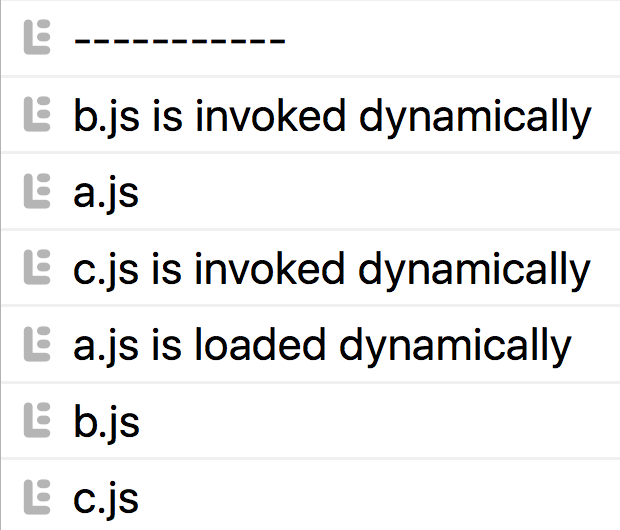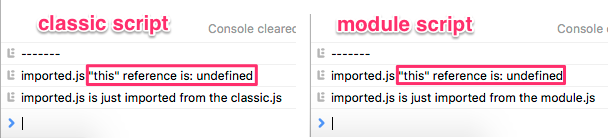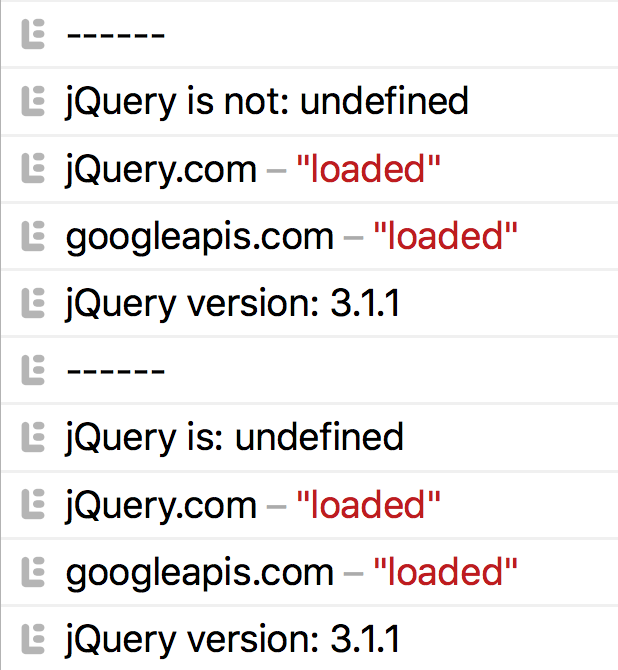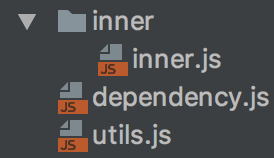• 裕波
• 9552 访问

# 语法

``````import("./specifier.js"); // 返回 Promise
``````

``````// STATIC
import './a.js';

import b from './b.js';
b();

import {c} from './c.js';
c();

// DYNAMIC
import('./a.js').then(()=>{
});

import('./b.js').then((module)=>{
const b = module.default;
b('isDynamic');
});

import('./c.js').then(({c})=>{
c('isDynamic');
});
``````

`'isDynamic'` 被传递过来区别于在模块中调用函数。下面是DEV控制台截图：```````<script type="module" src="static.js">`</script>
`<script src="dynamic.js">`</script>
``````

• 动态的 `import()` 提供一个基于Promise的API

• `import()` 遵循ES模块规则：singleton，说明符，CORS等.

• `import()` 可以在经典脚本和模块脚本中使用

• 在代码中使用的`import()`的顺序与它们被解析的顺序没有什么共同之处

# 脚本执行以及上下文

``````// imported.js
console.log(imported.js "this" reference is: \${this});
``````

``````<!--module.js-->
`<script type="module" src="module.js">`</script>

<!--classic.js-->
`<script src="classic.js">`</script>
``````
``````// module/classic.js
import('./imported.js').then(()=>{
console.log('imported.js is just imported from the module/classic.js');
});
``````# 额外特性

``````function loadUserPage(){
import('user-page.js').then(doStuff);
}

``````

``````// 加载脚本并且相应用户操作
FBshareBtn.on('click', ()=>{
import('/fb-sharing').then((FBshare)=>{
FBshare.do();
});
});
``````

``````const locale = 'en';
import(`./utils_\${locale}.js`).then(
(utils)=>{
console.log('utils', utils);
utils.default();
}
);
``````

``````if(user.loggedIn){
import('user-widget.js');
}
``````

• 您可以使用动态导入进行延迟或条件加载以及依赖用户的操作

• 动态的`import()` 可以在脚本的任何地方使用

• `import()`接受字符串文字，你可以根据你的需要构造说明符

## Promise API 优点

``````Promise.all([
import('./a.js'),
import('./b.js'),
import('./c.js'),
])
.then(([a, {default: b}, {c}]) => {

b('isDynamic');

c('isDynamic');
});
``````

``````const CDNs = [
{
name: 'jQuery.com',
url: 'https://code.jquery.com/jquery-3.1.1.min.js'
},
{
}
];

console.log(`------`);
console.log(`jQuery is: \${window.jQuery}`);

Promise.race([
]).then(()=> {
console.log(`jQuery version: \${window.jQuery.fn.jquery}`);
});
````````````// utils_en.js
const test = (isDynamic) => {
let prefix;
if (isDynamic) {
prefix = 'Static import';
} else {
prefix = 'Dynamic import()';
}

const phrase = `\${prefix}: ECMAScript dynamic module loader
"import()" works in this browser`;
console.log(phrase);
};

export {test};
``````
``````// STATIC
import {test} from './utils_en.js'; // no dynamic locale
test();

// DYNAMIC
(async () => {
const locale = 'en';

const {test} = await import(`./utils_\${locale}.js`);
test('isDynamic');
})();
``````

• 使用`Promise.all`并行加载模块

• 所有的Promise API 对于 `import()` 操作符都是可用的

• 您可以通过async/await来动态导入

## Promise API 警告

Here is a demo of importing a nonexisting script:

`````` import (`./non-existing.js`)
.then(console.log)
.catch((err) => {
console.log(err.message); // "Importing a module script failed."
// apply some logic, e.g. show a feedback for the user
});
``````

Here how you can add the global unhandled Promises listener:

``````window.addEventListener("unhandledrejection", (event)=> {
console.warn(`WARNING: Unhandled promise rejection. Reason: \${event.reason}`);
console.warn(event);
});
// process.on('unhandledRejection'... in case of Node.js
``````

# 警告

Let’s consider the following folder structure and the code:``````// utils.js - 用于加载依赖
export const loadDependency = (src) => {
return import(src)
.then((module) => {
return module;
})
};

// inner.js - 主文件将被用来测试传递的import()路径

// 加载资源失败,因为import()是在 ../dependency.js中调用

``````

• `import()` 说明符总是相对于调用它的文件

• 你可以在浏览器开发工具控制台使用动态导入 (对于调试很有帮助)

# 支持和polyfills

``````let dynamicImportSupported = false;
try{
Function('import("")');
dynamicImportSupported = true;
}catch(err){};

console.log(dynamicImportSupported);
``````

``````function importModule(url) {
return new Promise((resolve, reject) => {
const script = document.createElement("script");
Math.random().toString(32).substring(2);
script.type = "module";
script.textContent = `import * as m from "\${url}"; window.\${tempGlobal} = m;`;

resolve(window[tempGlobal]);
delete window[tempGlobal];
script.remove();
};

script.onerror = () => {
reject(new Error("Failed to load module script with URL " + url));
delete window[tempGlobal];
script.remove();
};

document.documentElement.appendChild(script);
});
}
``````

Babel为这种语法提供了dynamic-import-webpack插件，您可以安装它，并且`import()`操作符将由它处理。

Webpack 2支持使用动态`import()`开箱即用代码拆分，类似您以前使用的`require.ensure`

## 可用的importScripts(urls)

``````importScripts('foo.js', 'bar.js' /*, ...*/);
``````

# 最后

Webpack 2使用这个API，它目前在Stage 3上，已经在浏览器中实现，这意味着很快这个规范将成为一个标准。